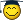# محاسبه حاصلضرب دو ماتریس و دترمینان ماتریس حاصلضرب در فرترن

#### زهرا گلمکانی

##### عضو جدید
PROGRAM GO
INTEGER ::I,J,M,N,S,K,G=2,T=0
REAL::w,DET=1
REAL,DIMENSION,,ALLOCATABLE::A
REAL,DIMENSION,,ALLOCATABLE::B
REAL,DIMENSION,,ALLOCATABLE::C
PRINT*,"ENTER M,N"
ALLOCATE(A(N,M))
ALLOCATE(B(M,N))
ALLOCATE(C(N,N))
PRINT*,"Enter A(", N ,",", M ,")"
PRINT*,"Enter B(",M,",",N,")"
DO I=1,N
DO J=1,N
C(I,J)=0
DO S=1,M
C(I,J)=C(I,J)+A(I,S)*B(S,J)
END DO
PRINT*,"C(",I,",",J,")=",C(I,J)
END DO
END DO
DO k=1,N
DO Q=1,N
SUM=ABS(C(K,Q))+SUM
END DO
IF(SUM==0) THEN
DET=0
ELSE
DO WHILE (C(K,K)==0)
T=T+1
DO I=1,N
C(I,K)=C(I,K)+C(I,K+T)
END DO
END DO
DO I=G,N
P=-C(I,K)/C(K,K)
DO J=1,N
C(I,J)=P*C(K,J)+C(I,J)
END DO
END DO
G=G+1
END IF
END DO
DO I=1,N
DET=C(I,I)*DET
END DO
PRINT*,"DET=",DET